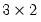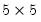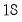Command Reference : Matrix Language Reference

 @zeros
Syntax: @zeros(n1, n2)
n1: integer
n2: integer
Return: matrix, vector
Creates a matrix or vector of the value 0. The size of the created matrix is given by the integers n1 (number of rows) and n2 (number of columns).
Examples
matrix m1 = @zeros(3,2)
creates M1, amatrix of zeros.
sym s1 = @zeros(5, 5)
sym s2 = @unvech(@zeros(5*6/2))
createsymmetric matrices of zeros, while
vector v1 = @zeros(18)
is anelement vector of zeros.
Cross-references